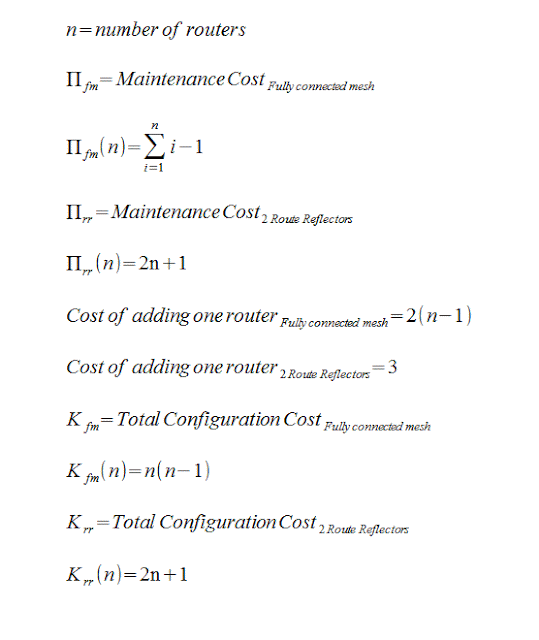## Pages

### BGP as IGP with next-hop-self RR vs Fully Connected Mesh

A comparison of BGP as iGP with next-hop-self in a fully connected mesh vs BGP as iGP with next-hop-self with two Route Reflectors.

This is an effort to figure out the best of the two setups in terms of configuration and maintenance cost and it is inspired by a quest in the AWMN mailing list to find the best setup for AWMN nodes with many routers .

( AWMN is a wireless BGP internet where each wireless node has an Autonomous System Number and 1 to 15 routers with wireless interfaces. The routing within each node is done with static Routes or some iGP --usually OSPF-- or iBGP with next-hop-self. )

I assume that:

The maintenance cost is equal to the number of iBGP sessions --the number of connections in the mesh.

The total configuration cost is equal to the number of (neighbor) configuration stanzas for all iBGP connections.

The cost of adding a router is equal to the number of (neighbor) iBGP configuration stanzas needed in all the nodes in the mesh.Get a little program that prints tables of maintenance and configuration costs for both setups.
```\$ wget https://raw.githubusercontent.com/ipduh/fmvsrr/master/fmvsrr.pl && chmod 755 fmvsrr.pl
```

Print costs for 2 to 27 routers.
```\$ ./fmvsrr.pl 27
N    = Number of routers
Πfm  = Maintenance Cost in a Fully Connected Mesh
Πrr  = Maintenance Cost in a Two Route Reflectors Setup
Kfm  = Total Configuration Cost in a Fully Connected Mesh
Krr  = Total Configuration Cost in a Two Route Reflectors Setup
Nfm  = Cost of adding one router in a Fully Connected Mesh
Nrr  = Cost of adding one router in a Two Route Reflectors Setup

N=2 Πfm=2  Πrr=2+  Kfm=2  Krr=2+  Nfm=2  Nrr=2+
N=3 Πfm=3  Πrr=3+  Kfm=6  Krr=3+  Nfm=6  Nrr=3
Ν=4  Πfm=6  Πrr=6  Kfm=12  Krr=9  Nfm=6  Nrr=3
Ν=5  Πfm=10  Πrr=7  Kfm=20  Krr=11  Nfm=8  Nrr=3
Ν=6  Πfm=15  Πrr=8  Kfm=30  Krr=13  Nfm=10  Nrr=3
Ν=7  Πfm=21  Πrr=9  Kfm=42  Krr=15  Nfm=12  Nrr=3
Ν=8  Πfm=28  Πrr=10  Kfm=56  Krr=17  Nfm=14  Nrr=3
Ν=9  Πfm=36  Πrr=11  Kfm=72  Krr=19  Nfm=16  Nrr=3
Ν=10  Πfm=45  Πrr=12  Kfm=90  Krr=21  Nfm=18  Nrr=3
Ν=11  Πfm=55  Πrr=13  Kfm=110  Krr=23  Nfm=20  Nrr=3
Ν=12  Πfm=66  Πrr=14  Kfm=132  Krr=25  Nfm=22  Nrr=3
Ν=13  Πfm=78  Πrr=15  Kfm=156  Krr=27  Nfm=24  Nrr=3
Ν=14  Πfm=91  Πrr=16  Kfm=182  Krr=29  Nfm=26  Nrr=3
Ν=15  Πfm=105  Πrr=17  Kfm=210  Krr=31  Nfm=28  Nrr=3
Ν=16  Πfm=120  Πrr=18  Kfm=240  Krr=33  Nfm=30  Nrr=3
Ν=17  Πfm=136  Πrr=19  Kfm=272  Krr=35  Nfm=32  Nrr=3
Ν=18  Πfm=153  Πrr=20  Kfm=306  Krr=37  Nfm=34  Nrr=3
Ν=19  Πfm=171  Πrr=21  Kfm=342  Krr=39  Nfm=36  Nrr=3
Ν=20  Πfm=190  Πrr=22  Kfm=380  Krr=41  Nfm=38  Nrr=3
Ν=21  Πfm=210  Πrr=23  Kfm=420  Krr=43  Nfm=40  Nrr=3
Ν=22  Πfm=231  Πrr=24  Kfm=462  Krr=45  Nfm=42  Nrr=3
Ν=23  Πfm=253  Πrr=25  Kfm=506  Krr=47  Nfm=44  Nrr=3
Ν=24  Πfm=276  Πrr=26  Kfm=552  Krr=49  Nfm=46  Nrr=3
Ν=25  Πfm=300  Πrr=27  Kfm=600  Krr=51  Nfm=48  Nrr=3
Ν=26  Πfm=325  Πrr=28  Kfm=650  Krr=53  Nfm=50  Nrr=3
Ν=27  Πfm=351  Πrr=29  Kfm=702  Krr=55  Nfm=52  Nrr=3

```

When the full mesh topology is used in a node with 10 routers the configuration and maintenance cost is ~4.5 times larger from a two-route-reflectors setup and the 11th router would cost me ~20 configuration stanzas and logging in 11 routers instead of ~3 stanzas in three routers ...

Full Mesh vs Route Reflectors